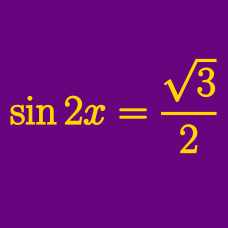Geometry

# Trigonometric Equations - R method

In the interval $$[0, 20 \pi ]$$, how many solutions are there to

$\sin \theta + \cos \theta = 1?$

What is the maximum value of the function $f(x)=2\sin \left(x+\frac{\pi}{3}\right)+\sqrt{3}\cos x+2 ?$

The sum of all the $$\theta$$'s in the interval $$12\pi < \theta < 14\pi$$ such that the quadratic equation for $$x$$ $x^2-2(\sin \theta+\cos \theta)x+\cos 2\theta=0$ has a repeated root is $$\frac{a}{2}\pi$$. What is the value of $$a?$$

If provided that $\sqrt{3}\cos \theta+\sin \theta=\frac{\sqrt{3}}{2}$ and $$\displaystyle \frac{\pi}{2} < \theta < \pi,$$ what is the value of $\sqrt{3}\sin \theta-\cos \theta ?$

What are the maximum and minimum values of the function $y=\sqrt{2}\sin x-\cos x ?$

×

Problem Loading...

Note Loading...

Set Loading...